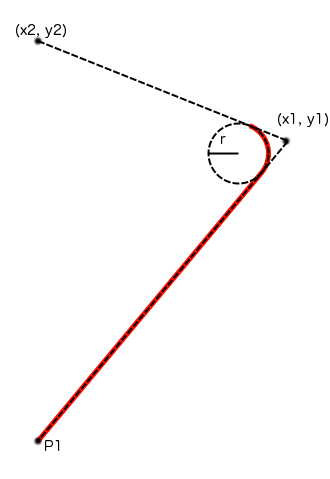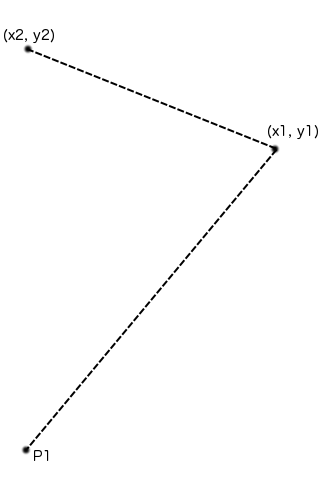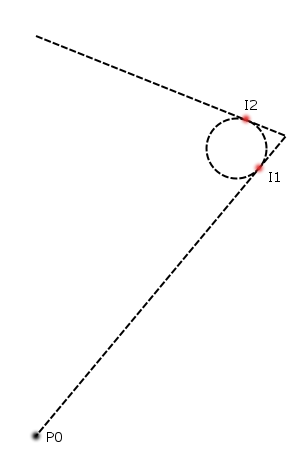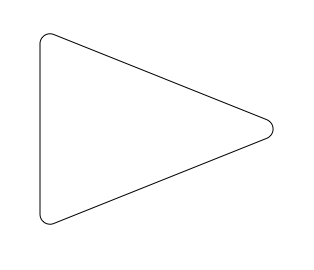CGPathAddArcToPoint and CGContextAddArcToPoint can be used easily to draw shapes with rounded corners. These two methods do exactly the same thing except that CGContextAddArcToPoint works on the path currently in a CGContext rather than a CGMutablePath.

Here is the function call for AddArcToPoint:

The function works like this:The red line is the path that the function will draw. P1 is the the point that the path is at before the function is called, x1, y1, x2, y2 correspond to the values passed in by the function, and r is the radius value. The function doesn’t line to the second point given, it stops at the end of the arc.

The way this is constructed is to imagine that you’ve drawn from the current point to the point at x1, y1 and then to x2, y2:Then you draw a circle with the given radius, and move it so that it just touches each of the lines:I1 and I2 are the points where the circle touches the lines.

addArcToPoint will make a path from the current point P0 to I1, it will then follow the arc of the circle around to I2 and then stop (It won’t make a path to the end point). This is shown in the initial diagram.

So you could implement a view to draw rounded triangles like this:

Here is a triangle drawn by the view above: